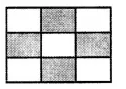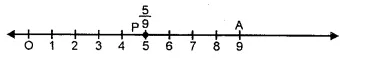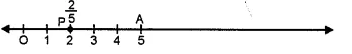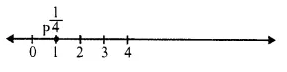## RS Aggarwal Class 6 Solutions Chapter 5 Fractions Ex 5A

These Solutions are part of RS Aggarwal Solutions Class 6. Here we have given RS Aggarwal Solutions Class 6 Chapter 5 Fractions Ex 5A.

Other Exercises

Question 1.
Solution:
(i) $$\\ \frac { 3 }{ 4 }$$
(ii) $$\\ \frac { 1 }{ 4 }$$
(iii) $$\\ \frac { 2 }{ 3 }$$
(iv) $$\\ \frac { 3 }{ 10 }$$
(v) $$\\ \frac { 4 }{ 9 }$$
(vi) $$\\ \frac { 3 }{ 8 }$$

Question 2.
Solution:
In the figure, $$\\ \frac { 4 }{ 9 }$$ is shadedQuestion 3.
Solution:
In the figure, whole rectangle is not divided into four equal parts.

Question 4.
Solution:
(i) Three-fourths = $$\\ \frac { 3 }{ 4 }$$
(ii) Four-sevenths = $$\\ \frac { 4 }{ 7 }$$
(iii) Two-fifths = $$\\ \frac { 2 }{ 5 }$$
(iv) Three-tenths = $$\\ \frac { 3 }{ 10 }$$
(v) One-eighth = $$\\ \frac { 1 }{ 8 }$$
(vi) three-tenths = $$\\ \frac { 5 }{ 6 }$$
(vii) five-sixths = $$\\ \frac { 8 }{ 9 }$$
(vii) seven-twelfths = $$\\ \frac { 7 }{ 12 }$$

Question 5.
Solution:
(i) In $$\\ \frac { 4 }{ 9 }$$, numerator is 4 and denominator is 9.
(ii) In $$\\ \frac { 6 }{ 11 }$$, numerator is 6 and denominator is 11.
(iii) In $$\\ \frac { 8 }{ 15 }$$, numerator is 8 and denominator is 15.
(iv) In $$\\ \frac { 12 }{ 17 }$$, numerator is 12 and denominator is 17.
(v) $$\\ \frac { 5 }{ 1 }$$ , numerator is 5 and denominator is 1.

Question 6.
Solution:
(z) Numerator = 3, Denominator = 8, then fraction = $$\\ \frac { 3 }{ 8 }$$.
(ii) Numerator = 5, Denominator = 12, then fraction = $$\\ \frac { 5 }{ 12 }$$
(iii) Numerator = 7, Denominator = 16, then fraction = $$\\ \frac { 7 }{ 16 }$$.
(iv) Numerator = 8, Denominator = 15, then fraction = $$\\ \frac { 8 }{ 15 }$$

Question 7.
Solution:
(i) $$\\ \frac { 2 }{ 3 }$$ = two-thirds
(ii) $$\\ \frac { 4 }{ 9 }$$ = four-ninths
(iii) $$\\ \frac { 2 }{ 5 }$$ = two-fifths
(iv) $$\\ \frac { 7 }{ 10 }$$ = seven-tenths
(v) $$\\ \frac { 1 }{ 3 }$$ = one-thirds
(vi) $$\\ \frac { 3 }{ 4 }$$ = three-fourth
(vii) $$\\ \frac { 3 }{ 8 }$$ = three-eighths
(viii) $$\\ \frac { 9 }{ 14 }$$ = nine-fourteenths
(ix) $$\\ \frac { 5 }{ 11 }$$ = five-elevanths
(x) $$\\ \frac { 6 }{ 15 }$$ = six-fifteenths

Question 8.
Solution:
24 minutes is the fraction of 1 hour i.e.,
60 minutes = $$\\ \frac { 24 }{ 60 }$$

Question 9.
Solution:
Natural number between 2 to 10 are 2, 3, 4, 5, 6, 7, 8, 9, 10 = 9
Out of these prime number are 2, 3, 5, 7 = 4
Fraction = $$\\ \frac { 4 }{ 9 }$$

Question 10.
Solution:
(i) $$\\ \frac { 2 }{ 3 }$$ of 15 pens = $$\\ \frac { 2 }{ 3 }$$ x 15 = 2 x 5 = 10 pens.
(ii) $$\\ \frac { 2 }{ 3 }$$ of 27 balls = $$\\ \frac { 2 }{ 3 }$$ x 27 = 2 x 9 = 18 balls.
(iii) $$\\ \frac { 2 }{ 3 }$$ of 36 balloons = $$\\ \frac { 2 }{ 3 }$$ x 36 = 2 x 12 = 24 balloons. Ans.

Question 11.
Solution:
(i) $$\\ \frac { 3 }{ 4 }$$ of 16 cups = $$\\ \frac { 3 }{ 4 }$$ x 16 = 3 x 4
= 12 cups.
(ii) $$\\ \frac { 3 }{ 4 }$$ of 28 rackets = $$\\ \frac { 3 }{ 4 }$$ x 28 = 3 x 7
= 21 rackets.
(iii) $$\\ \frac { 3 }{ 4 }$$ of 32 books = $$\\ \frac { 3 }{ 4 }$$ x 32 = 3 x 8
= 24 books. Ans.

Question 12.
Solution:
Total number of pencils Neelam has = 25
No. of pencils given to Meena
= $$\\ \frac { 4 }{ 5 }$$ of 25
= $$\\ \frac { 4 }{ 5 }$$ x 25 – 20
No. of pencils left with Neelam = 25 – 20 = 5

Question 13.
Solution:
(i) $$\\ \frac { 3 }{ 8 }$$
Take a line segment OA = one unit of length
Divide it into 8 equal parts and take 3 parts at P, then P represents $$\\ \frac { 3 }{ 8 }$$.(ii) $$\\ \frac { 5 }{ 9 }$$
(a) Take a line segment OA = one unit of length.
(b) Divide it into nine equal parts and take 5 parts at P, then P represents $$\\ \frac { 5 }{ 9 }$$.(iii) $$\\ \frac { 4 }{ 7 }$$
(a) Take a line segment OA = one unit of length.
(b) Divide it into 7 equal parts and take 4 parts at P then P represents $$\\ \frac { 4 }{ 7 }$$.(iv) $$\\ \frac { 2 }{ 5 }$$
(a) Take a line segment OA = 1 unit of length.
(b) Divide it with 5 equal parts and take 2 parts and P then P represents $$\\ \frac { 2 }{ 5 }$$.(v) $$\\ \frac { 1 }{ 4 }$$
(a) Take a line segment OA = 1 unit of length.
(b) Divide it with 4 equal parts and take 1 parts and P then P represents $$\\ \frac { 1 }{ 4 }$$.Hope given RS Aggarwal Solutions Class 6 Chapter 5 Fractions Ex 5A are helpful to complete your math homework.

If you have any doubts, please comment below. Learn Insta try to provide online math tutoring for you.